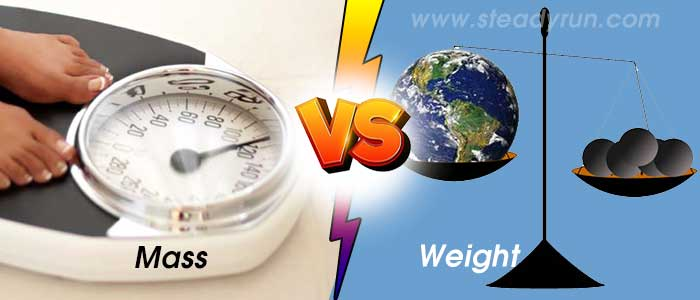# Difference Between Mass and WeightDistinguish, differentiate, compare and explain what is the differences between mass and weight for kids in science, physics, and chemistry with examples. Comparison and Differences.

### What do you mean by mass ?

Mass is the amount of matter in an object. It can also be defined as the property of a body that causes it to have weight in a gravitational field. It is important to understand that the mass of an object is not dependent on gravity. Bodies with greater mass are accelerated less by the same force.

### What do you mean by weight?

Weight is the vertical force exerted by a mass as a result of gravity. Weight can also be defined as the strength of the gravitational pull on the object; that is, how heavy it is. Weight is dependent on gravity. Using more physics and mathematics terms, weight is defined as the force with which a body is attracted to Earth or another celestial body, equal to the product of the objects mass and the acceleration of gravity: gravity = mass x acceleration due to gravity, which is constant (Earth = 9.8 m/sec2 ).

## Difference between Mass and Weight

 S.No. Mass Weight 1 It is a property of matter and it remains same everywhere. It is denoted using m or M. It depends on the effect of gravity. It varies according to location. It is equal to mass multiplied by the acceleration of gravity. W = m * g 2 According to location, the mass of an object or body remains constant. Weight increases or decreases according to the gravity acting on it. 3 It can never be zero. It can be zero if no gravity acts upon an object. For Example, in space. 4 Scalar quantity; it has magnitude. Vector quantity, it has both magnitude and direction, it is directed toward the center of the Earth or other gravity well. 5 Units are grams and kilograms. The unit is Newtons, a unit of force. 6 May be measured using an ordinary balance. It is measured using a spring balance.

### Weight vs. Mass

There is often confusion about the terms weight and mass. This is a short description of these two terms.

Weight is a force. It is the amount of pulling force that gravity exerts on an object. What is really interesting is the amount of force acting on an object causes that object to accelerate at -9.8 m/s2 (if that is the only force acting on the object). Since weight is a force, it has units of Newtons (SI units). However, most of us are more used to units of pounds or lbs because we all step on a scale and see weight in lbs.

The equation for weight looks like this:
FGravity = mg

Where g is -9.8 m/s/s and represents the acceleration due to the force of gravity and m represents the objects mass.

Mass is a measure of an objects inertia. Inertia is a mechanical term used to describe an objects resistance to change motion. That means that the more inertia an object has, the harder it is to get going (from a stopped position) and the harder it is to stop the object from moving. We already know this. Can you imagine kicking a soccer ball vs. kicking a bowling ball?! Of course we would not kick a bowling ball. It has too much inertia (too much resistance to change motion) and it would take too much force to change the motion (and the foot would likely be injured!).

To determine the mass of the object, we would simply take the objects weight and divide by the acceleration due to the force of gravity. The equation looks like this: m = FGravity/g. Remember, the force of gravity is negative (since it is pulling down on the object) and the acceleration due to the force of gravity is also negative; therefore, mass is positive.

#### Tags:

Difference between Weight vs Difference Between Mass

Difference Between Mass vs Weight

Differences between Weight vs Difference Between Mass

## Related Categories### Spreading Knowledge Across the World

USA - United States of America  Canada  United Kingdom  Australia  New Zealand  South America  Brazil  Portugal  Netherland  South Africa  Ethiopia  Zambia  Singapore  Malaysia  India  China  UAE - Saudi Arabia  Qatar  Oman  Kuwait  Bahrain  Dubai  Israil  England  Scotland  Norway  Ireland  Denmark  France  Spain  Poland  and  many more....